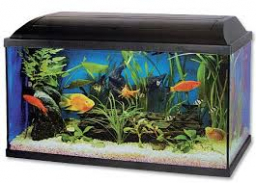# Calculate 64644

The aquarium has a length of 5dm and a width of 4dm and contains 60 liters of water.
A) calculate the height of the aquarium if the water reaches 5 cm below the edge
B) calculate the total volume of the aquarium

c =  35 cm
V =  70 l

### Step-by-step explanation:Did you find an error or inaccuracy? Feel free to write us. Thank you!

Tips for related online calculators
Need help calculating sum, simplifying, or multiplying fractions? Try our fraction calculator.
Do you know the volume and unit volume, and want to convert volume units?